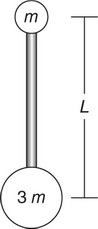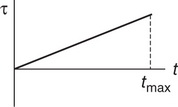# AP Physics 1 Practice Test 20

### Test Information10 questions18 minutes

1.Question below refers to the following information:

Block B is at rest on a smooth tabletop. It is attached to a long spring, which in turn is anchored to the wall. Identical block A slides toward and collides with block B. Consider two collisions, each of which occupies a duration of about 0.10 s:

Collision I: Block A bounces back off of block B.

Collision II: Block A sticks to block B.

In which collision, if either, is the period and frequency of the ensuing oscillations after the collision larger?

2. A string is fixed at one end but free to move at the other end. The lowest frequency at which this string will produce standing waves is 10 Hz. Which of the following diagrams represents how the string will look when it is vibrating with a frequency of 30 Hz?

3.Three equal-mass objects (A, B, and C) are each initially at rest horizontally on a pivot, as shown in the figure. Object A is a 40 cm long, uniform rod, pivoted 10 cm from its left edge. Object B consists of two heavy blocks connected by a very light rod. It is also 40 cm long and pivoted 10 cm from its left edge. Object C consists of two heavy blocks connected by a very light rod that is 50 cm long and pivoted 20 cm from its left edge. Which of the following correctly ranks the objects’ angular acceleration about the pivot point when they are released?

4.A stationary wave source emits waves at a constant frequency. As these waves move to the right, they are represented by the wave front diagram shown here. Sometime later, the wave source is moving at a constant speed to the right. Which of the following wave front diagrams could represent the propagation of the waves produced by the source?

5.An object of mass m is attached to an object of mass 3m by a rigid bar of negligible mass and length L. Initially, the smaller object is at rest directly above the larger object, as shown in the figure. How much work is necessary to flip the object 180°, such that the larger mass is at rest directly above the smaller mass?

6. Which of the experiments listed here measure inertial mass? Select two answers.

7. An object on a spring vibrates in simple harmonic motion. A sonic motion detector is placed under the object. Which of the following determines the period of the object’s oscillation? Select two answers.

8. Two carts on a negligible-friction surface collide with each other. Which of the following is a correct statement about an elastic collision between the carts? Select two answers.

9. The resistance of a sample of circular cross-section wire is known. A measurement of which two of the following would allow for a calculation of the resistivity of the wire? Select two answers.

10.The net torque τ on an object of rotational inertia I is shown as a function of time t. At time tmax, the object has speed ω and angular acceleration α. Which of the following methods correctly determines the change in the object’s angular momentum? Select two answers.